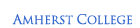IT > Software > Excel Tutorials > Analyzing Data with Excel# Analyzing Data with Excel

### Author: Andy AndersonDate: June 2007

Excel provides a substantial collection of tools for analyzing data, some of which will be demonstrated in this tutorial.

Those who are unfamiliar with Excel may wish to first read The Basics of Using Excel.

The fundamentals of graphing data with Excel are described in Graphing with Excel.

Those interested in another application of Excel in the curriculum should read Grading with Blackboard and Excel.

## Importing Text Data

Your data will often come in plain text formats, which Excel can import without too much trouble.

Data that you want to analyze will often be in one of several formats, with plain text being very common.

Text files that have data in rows and using commas to delimit or separate columns, e.g.

Year,Jan,Feb,Mar,Apr,May,Jun,Jul,Aug,Sep,Oct,Nov,Dec,J-D,D-N,DJF,MAM,JJA,SON,Year 1880,28,10,-9,-15,-12,-55,-21,3,8,-39,-33,-3,*********,*****,-12,-25,-21,1880 1881,-54,-3,-15,8,21,-86,-5,-8,-14,-17,-17,27,-14,-16,-20,4,-33,-16,1881 ....

can be given the file extension .CSV (Comma-Separated Values), and Excel will immediately know what to do with them.

As an example, download the file

and try opening it from within Excel.

Text files may also use tab characters to delimit columns.

This can make the file slightly more readable but columns won't necessarily line up due to varying data lengths.

As an example, download the file

When you open this file from within Excel, it will display the Text Import Wizard to guide you through the process: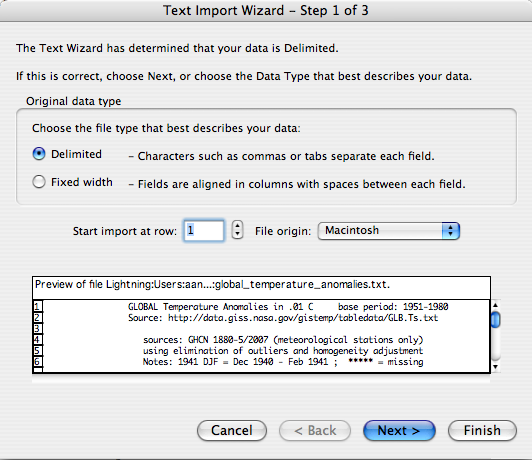This is pretty straightforward as long as you verify in the next step that the delimiter is the tab: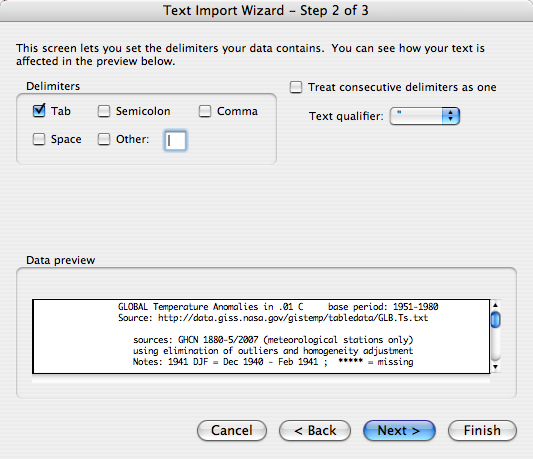In the final stage you can choose the data type; as in this file the data is often mixed, in which case General is the best option: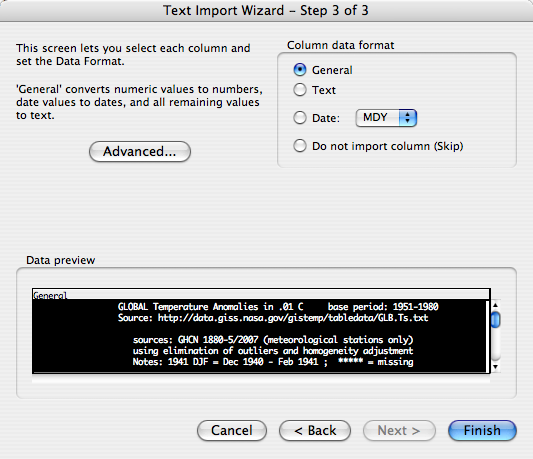Afterward you can choose data types on a column-by-column basis.

You may also sometimes run into data that is fixed-width, meaning that every column starts at the same number of characters from the beginning of the row.

The Text Import Wizard shows this option in the first of the previous three images, but it won't be discussed for now.

After importing text data, you'll want to save it in Excel format to avoid complicating behaviors of Excel:

 Menu: File (Office)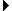Save As... Navigate: (to wherever you want to save the file) Menu: Format: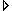Excel Workbook (*.xlsx) or                  Excel 97-2003 Workbook (*.xls) (for backward compatibility) Click: Save

You'll also often need to clean such files up.

For this example, there are two things to do in the above data set:

1. Delete lines that break the data up, such as the extra column headers;
2. Change the "*****" cells to blank cells, which is how Excel handles missing data.

## Linear Data Fitting

Excel provides a limited set of tools for fitting data to a linear combination of expressions.

One of the nice things you can quickly do with Excel is add a linear fit to any data that you graph, and get basic statistics from it immediately.

The data that you imported in the previous section is the global temperature anomaly from 1880 onward, expressed as a deviation in hundredths of degrees Celsus from a reference temperature, 14 °C. The column headed J-D is the average for the entire year.

Using your previous knowledge of Graphing with Excel, graph J-D versus the year using an XY chart; your result should look something like the following: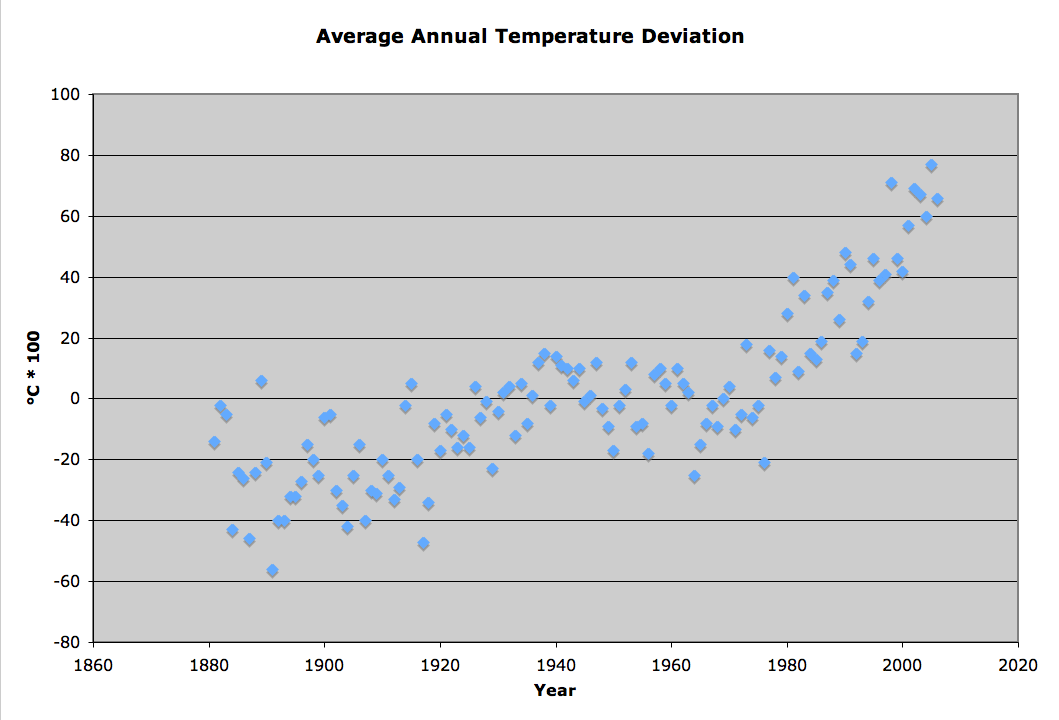We can now add a trend line to this chart:

 Click: the data of interest Menu: Chart ToolsLayoutAnalysisTrendline Click: More Trendline Options… Click: Format TrendlineLinear Click: Display Equation on chart Click: Display R-squared value on chart Click: Close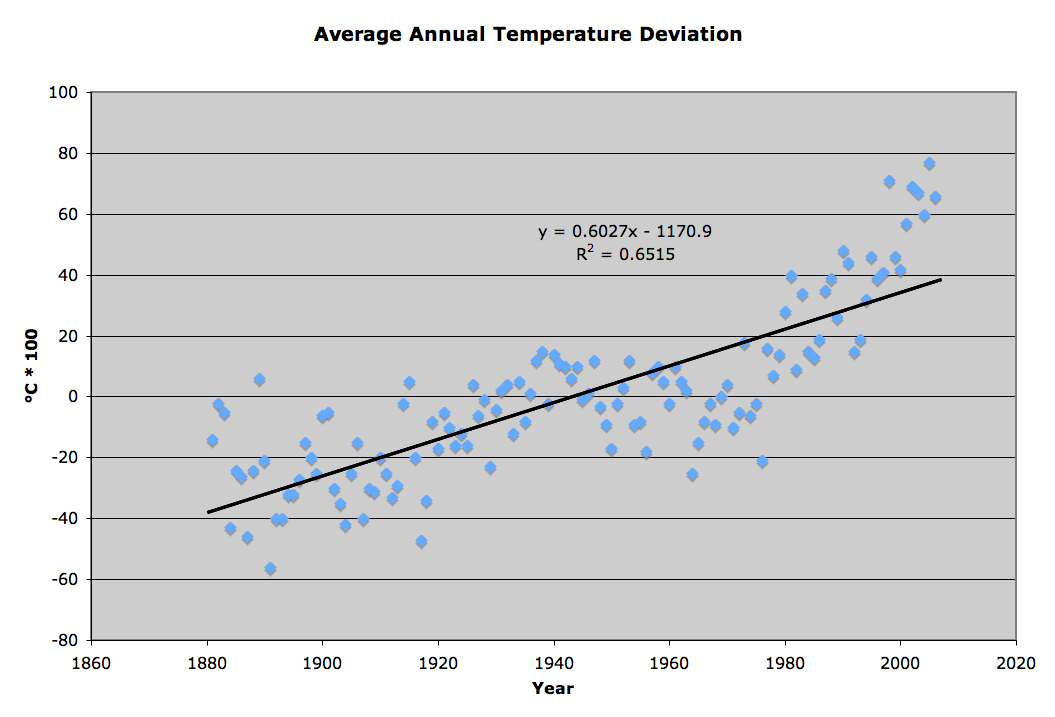R2 is called the Coefficient of Determination. According to the Excel documentation, it "compares estimated and actual y-values, and ranges in value from 0 to 1. If it is 1, there is a perfect correlation in the sample — there is no difference between the estimated y-value and the actual y-value. At the other extreme, if the coefficient of determination is 0, the regression equation is not helpful in predicting a y-value."

You may noticed the other options in the Type page of the Trendline dialog, e.g. Exponential, Polynomial, etc. which are basically linear fits to functional transformations of your data.

Question: What would be another good functional form to try, given what this data represents? What would you have to do to the data to make it work in this case?

You can add multiple trend lines to your graph if you want, and change their colors and patterns by double-clicking on them.

Excel also provides a more general linear data-fitting feature that provides all of the details of the statistical fit:

 Menu: DataAnalysisData Analysis Click: Data AnalysisRegression Click: OKAt a minimum you must fill in the fields Input Y range: and Input X range: (in this case with just the data under Year and J-D).

Note that the ranges the dialog expects must be the same length, cannot include headers, and cannot begin or end with blank cells.

It's simplest to accept the default output range — New worksheet ply: — so that you will be sure to avoid overwriting your data if your output range isn't selected carefully.

An Excel workbook can contain multiple sheets of data; switch between them by clicking on the tabs at the lower left: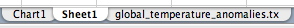Your result will look something like the following, depending on which options you request in the dialog above:You'll notice the R Square and Coefficients values, which are the same as those plotted above; in addition, you'll see the standard error on the coefficients, as well as the Standard Error for values calculated with the equation.

Any statistics book should explain what the other values represent.

## Solutions of Equations

Excel can also perform some iterative solution finding.

A common data analysis procedure is searching for solutions of equations, such as finding the zero-value of the equation above:

y = 0.6027 x - 1170.9

Since this is linear, we can make the solution somewhat less obvious by using a quadratic fit instead:

y = 0.0058 x2 - 22.041 x + 20919

In Excel, this equation is expressed through two cells, one that holds an independent variable x (A1 in the picture below), and one that calculates the dependent variable y using the functional relation (B1 in the picture):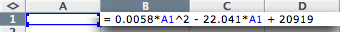To find the solution y = 0, you could try out different values in the cell B1 until you approach a value of zero in cell A1, or you could let Excel perform this iterative process for you with its Solver:

 Click: whichever cell holds the dependent variable y Menu: DataAnalysisSolver...At a minimum you must fill in the following items in this dialog:

1. Set Target Cell: This should be a reference to the cell holding the calculation of the dependent variable; Excel initially sets it to whichever cell is selected.
2. Equal To: This describes the type of solution you are looking for in the Target Cell, in this case Value of: 0. Note that you can search for any particular value, along with maximums and minimums.
3. By Changing Cells: This is the cell (or range of cells) holding the independent variable(s) that will be iteratively changed to find the solution. Excel initially sets it to whichever cell(s) are referenced by the initial Target Cell. You can also click on the button Guess, and Excel will reanalyze the Target Cell.

Commonly you will also need to set constraints on your solutions. For example, with the quadratic equation used here, there are two places where it has the value y = 0, so we can limit the search range to find the one we want:

 Click: Solver ParametersSubject to the ConstraintsAdd Click: Add ConstraintCell ReferenceIndependent Variable Cell Menu: Relationship Type: ConstraintValue Click: OK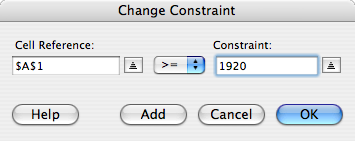It can also help to provide an approximate value in the independent variable cell(s).

Finally, to find the the solution,

 Click: Solver ParametersSolve Click: OK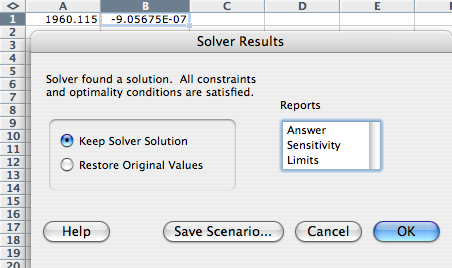The reports provided here can describe some of the issues involved in finding the solution; click and shift-click to select them.

If you have problems finding a solution, you may need to delve into some of the details of the iterative process.

In the Solver dialog, the button Options... provides some control over the iteration method used.

The option Show Iteration Results can be particularly useful to step through the iteration and figure out how it might be going wrong.

## Pivot Tables

A lot of data you will find has a "tree" structure, where a lot of the information is the same from row to row; pivot tables help to group this information.

The following data set lists all of the counties in the USA, grouped by the state they are in:

Top of Page  | Using IT DocumentationIT Home  |  IT Site Map  |  Search IT